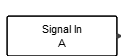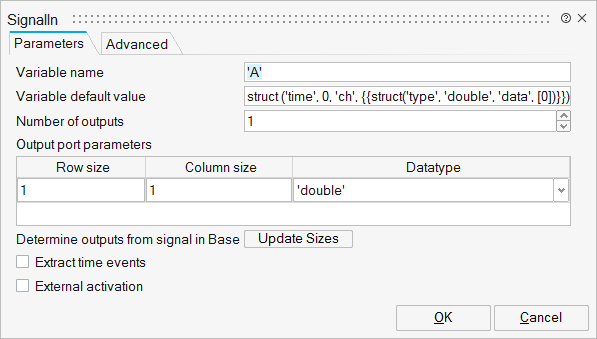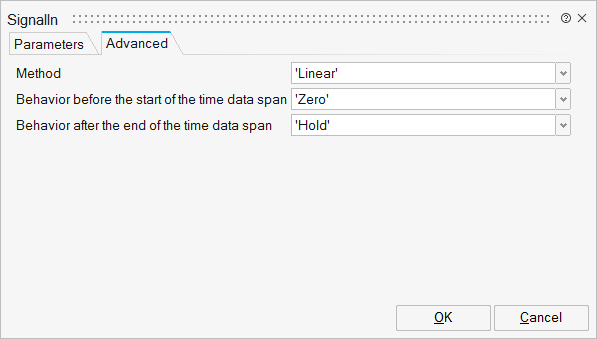# SignalIn

This block takes variables defined in the OML workspace and treats them as signals. The variable should have "time" and "ch" fields.SignalImporters

## Description

This block is used to get variables defined in the OML workspace and use them in Activate.

The variable can be defined either in the OML shell or by a SignalOut block.

For example, the following OML command creates a variable B:

B=struct ("time", [0,1,3], "ch", {{struct("type", "double", "data", [2,5,3])}})

The Variable B defines the value of a signal at three instants 0, 1, and 3. A variable can be of any datatype supported by Activate.

A SignalOut block is usually used to create a variable acceptable by the SignalIn block. The SignalIn block can reconstruct the original signal fed to the SignalOut block in another model or simulation.

The variable provides the values of a signal V(i), at different time instants T(i). The value of the signal at times other than elements of T(i) are computed via interpolation or extrapolation. Several interpolation methods are available:"ZeroOrder", "Linear", "Not_a_knot", "Natural", and "Clamped_To_Zero".

"ZeroOrder": The element in T(i) which is the nearest and below the current time is gievn at the output, i.e.,"Linear". This is the default method and performs a linear interpolation. If the time matches one of T elements, the output is the corresponding element in the V matrix. If no value matches the block's input, then the block performs linear interpolation between the two appropriate elements of the table to determine the output value, i.e.,"Not_a_knot": A cubic spline is computed and used by imposing the following conditions (considering N points T(1),...,T(N)) :"Natural": A cubic spline is computed and used by imposing the following conditions (considering N points T(1),...,T(N)) :"Clamped_To_Zero": A cubic spline is computed and used by imposing the following conditions (considering N points T(1),...,T(N)) :The value of the signal at time instants out of the range [T(1), T(N)] are computed via extrapolation. Several extrapolation methods are available: "Zero", "Hold", "Extrapolation", and "Repeat".

"Zero":This is the default method. In this case, the output is zero for time instants out of signal's range, i.e.,"Hold": The output is equal to the last element of the V vector, i.e.,"Extrapolation". The block performs linear extrapolation using two last elements of the signal, i.e.,"Repeat". The signal is repeated over the whole range.The variable should have "time" (time instants vector) and "ch" (channels) fields and the "ch" field should have "type" (datatype) and "data" (data values) fields.

If the variable is not defined in the OML workspace, the default value of the variable is used instead. The default value is a parameter of the block.

## ParametersNameLabelDescriptionData TypeValid Values

varnam

Variable name

Name of the variable in the OML workspace.

String

defvalue

Variable default value

Value used in case the variable is not defined in the OML workspace.
Default is set to struct('time',0,'ch',{struct('type','double','data',)})

nout

Number of outputs

Number of outputs, equal to the number of channels in the cell "ch".

Number

outports

Output port parameters

Structure

outports/outrow

Row size

Cell of scalars

outports/outcol

Column size

Cell of scalars

outports/ot

Datatype

Cell of strings

'double'
'complex'
'int32'
'int16'
'int8'
'uint32'
'uint16'
'uint8'
'boolean'
'inherit'

outevent

Extract time events

This parameter defines if the block generates an activation event. When OutEvent is used, a additional event output port is added to the block. default is OFF.

Scalar

0
1

externalActivation

External activation

This parameter defines if the block receives an external activation or inherits. When External Activation is used, a additional activation port is added to the block. default is OFF.

Number

0
1NameLabelDescriptionData TypeValid Values

Method

Method

String

'ZeroOrder'
'Linear'
'Not_a_knot'
'Natural'
'Clamped_To_Zero'
'Akima'
'Fritsch_Butland'
'Steffen'

Leftside

Behavior before the start of the time data span

String

'Zero'
'Hold'
'Extrapolation'
'Repeat'

Rightside

Behavior after the end of the time data span

String

'Zero'
'Hold'
'Extrapolation'
'Repeat'

## Ports

NameTypeDescriptionIO TypeNumber

Port 1

explicit

Output variable value.

output

nout

Port 2

activation

External activation signal. If the block is activated by ths port, the time vector of the workspace signal is ignored.

input

externalActivation

Port 3

activation

If this port is displayed, it generates events at time instants of the vector T(i). This event is generated if ZeroOrder and Linear interpolation methods are chosen.

output

outevent

NameValueDescription

always active

no

Time Dependency = standard mode activated

direct-feedthrough

no

zero-crossing

no

mode

no

continuous-time state

no

discrete-time state

no